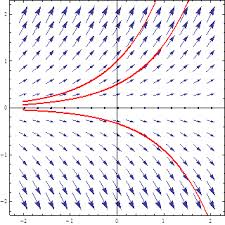# Zelik paper accepted for publication in J Math PhysicsThe paper “Global solvability and blow up for the convective Cahn-Hilliard equations with concave potentials“, by A Eden (Bogazici University, Turkey), V.K. Kalantarov (Koc University, Turkey) and Sergey Zelik, has been accepted for publication in the Journal of Mathematical Physics.  The paper studies initial boundary value problems for the unstable convective Cahn-Hilliard (CH) equation, i.e., the Cahn Hilliard equation whose energy integral is not bounded below.  It is well-known that without the convective term, the solutions of the unstable CH equation may blow up in finite time. In contrast, this paper shows that the presence of a convective term in the Cahn-Hilliard equation prevents blow up. It is also shown that the blowing up solutions still exist if the exponent is large enough.  Related equations, like the Kolmogorov-Sivashinsky-Spiegel equation, and the sixth order convective Cahn-Hilliard equation, are also considered.  The link to the paper on the JMP website is here.